## Thursday, December 31, 2020

### Silent Reflections

Last comic of 2020. We're all glad to see it go. And for now, we'll pretend that tomorrow everything will be all better.

Update: Happy New Year to my readers. And I'm happy to see that some of you still get the RSS feed, as you've gotten to this comic without my linking it anywhere. Notes below:

(Click on the comic if you can't see the full image.)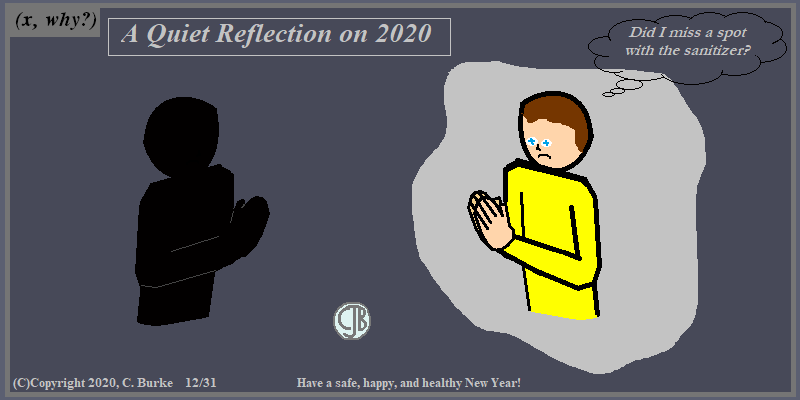(C)Copyright 2020, C. Burke. "AnthroNumerics" is a trademark of Christopher J. Burke and (x, why?).

Just a Silent Reflection

While I wouldn't put it past me to have published a blank blog post to sum up my feelings for the year, the comic above should have appeared Sunday or Monday. I thought it would be a good one for this past week between Christmas and New Years. But life and other things have a way of interfering with plans. (Nothing bad, just busy-ness. Not even "business".)

Funny thing, I wasn't going to fix the post on New Years Eve because I was spending time with the family. And I'm always up early anyway.

But it's a new year, and my wife opened a cluttered kitchen cabinet. And since New Years are for New Starts and all that, well, let's say that it's six cabinets later, and here's the update on New Years Day.

I hope everyone had a happy, healthy, and safe New Year.

Come back often for more funny math and geeky comics.## Friday, December 25, 2020

### What Social Media Christmas Comic is This?

(Click on the comic if you can't see the full image.)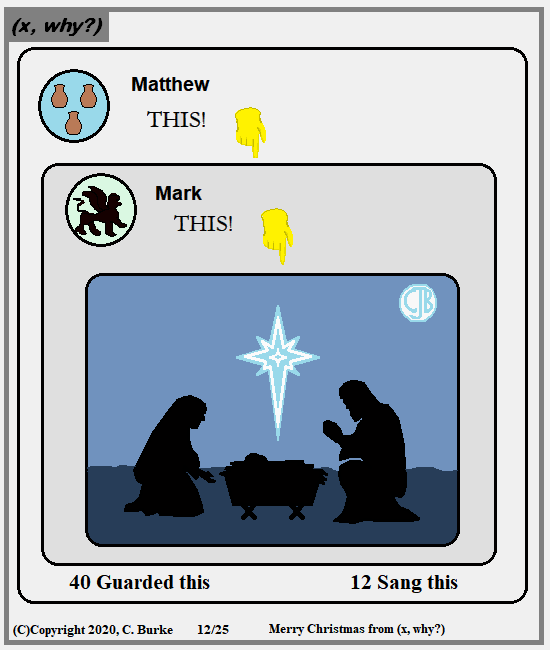(C)Copyright 2020, C. Burke. "AnthroNumerics" is a trademark of Christopher J. Burke and (x, why?).

Merry Christmas 2020! Share the Joy!

It's been an interesting year, to say the least. And it ended with several projects going on, several irons in the fire, as it were, and there just wasn't enough time.

Amusingly, the one comic that I had written out from 2019 had to be cut because of two surprise family gatherings of sorts yesterday. (And even without that, it was iffy.) But, on the bright side, I have a comic idea ready to go in December 2021!

Come back often for more funny math and geeky comics.## Wednesday, December 23, 2020

### Pre-Christmas Problems

(Click on the comic if you can't see the full image.)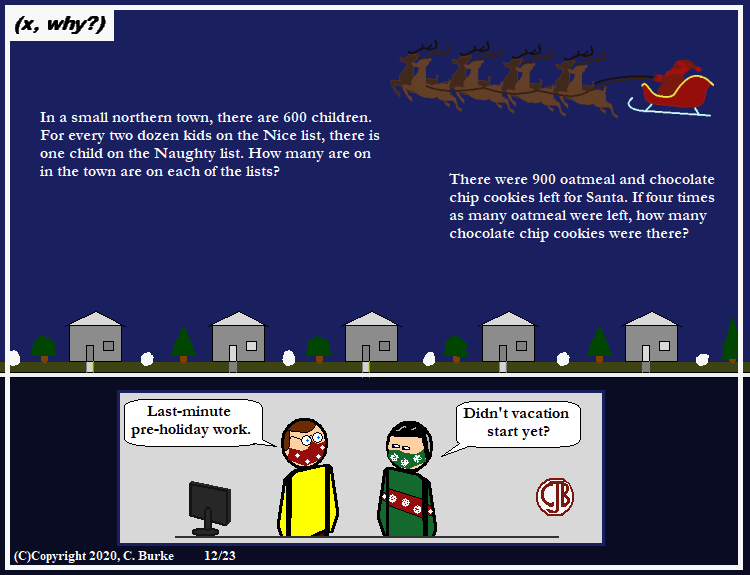(C)Copyright 2020, C. Burke. "AnthroNumerics" is a trademark of Christopher J. Burke and (x, why?).

You're not starting a new topic. And youre probably not really reviewing any old topics either.

In years past, my love for this time of year usually shines through. This year? Too many irons on the fire? Maybe a little uninspired? I don't know. I've had thoughts written down, but creating them doesn't always seem to happen.

On the other hand, I'm happy with how my reindeer and sled came out. Yes, they're based on clip art images, but they are my own. And there will be one, maybe two more Christmas comics by Friday. I hope.

Come back often for more funny math and geeky comics.## Friday, December 18, 2020

### Happy Pills

(Click on the comic if you can't see the full image.)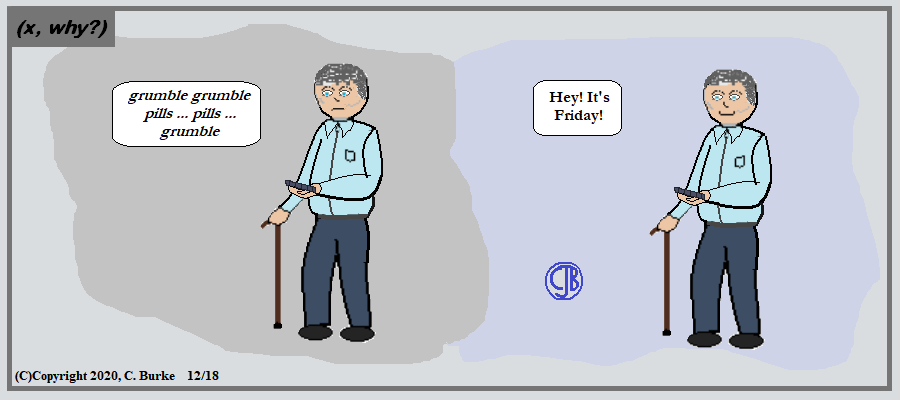(C)Copyright 2020, C. Burke. "AnthroNumerics" is a trademark of Christopher J. Burke and (x, why?).

Especially under lockdown, it's easy to forget what day it is.

I know I haven't started any Christmas/Holiday comics yet. I just haven't been inspired, even with the recent snow storm. This year is crazy, and worse is knowing that it won't all magically change on January 1.

Come back often for more funny math and geeky comics.## Wednesday, December 16, 2020

### Coffee Cup Day!

(Click on the comic if you can't see the full image.)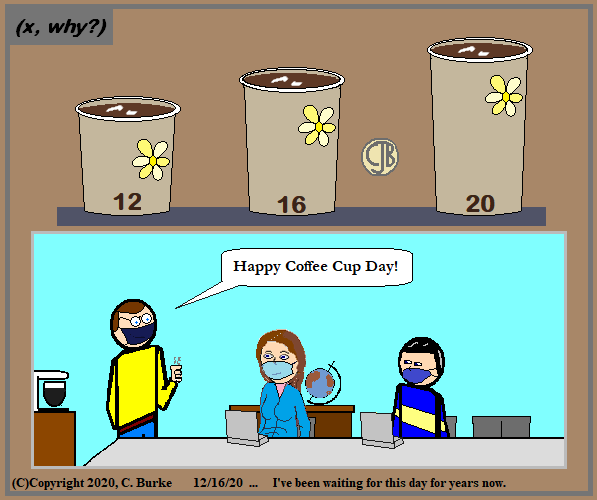(C)Copyright 2020, C. Burke. "AnthroNumerics" is a trademark of Christopher J. Burke and (x, why?).

Thank you, come again. Maybe on 12-20-24.

I have been waiting for this for years because the lids on the coffee cups -- which haven't changed despite the cups changing -- say 12 - 16 - 20 on them. They fit the most common sizes sold in grocery stores and delis. Most of those places don't sell 24 oz coffee (or larger) like some of the chain stores do. However, sometimes the larger cups have different size lids.

Come back often for more funny math and geeky comics.## Tuesday, December 15, 2020

### School Life #19: Hero Props

(Click on the comic if you can't see the full image.)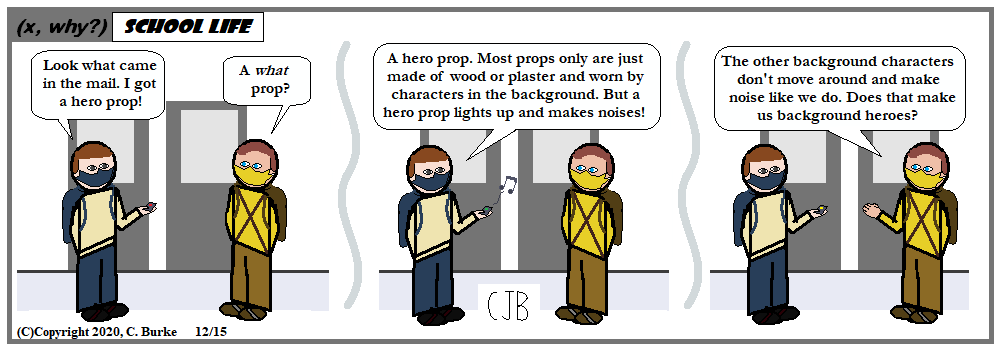(C)Copyright 2020, C. Burke. "AnthroNumerics" is a trademark of Christopher J. Burke and (x, why?).

Maybe they're heroes for hire. They're around when I need them. And they apparently have Comic Self-Awareness.

And, as stated on the Character Page, they've not only had lines, but have delivered the punch lines of strips in which they appeared. The Character Page is relatively new, and already a little out of date. One thing at a time.

I learned about hero props at a panel at I-Con Stony Brook many, many years ago. The fellow running the panel, in a classroom at the college, had a bunch of stage props and a few hero props, mostly from Star Trek and Battlestar Galactica -- the original. This was before the reboot existed. In fact, Richard Hatch was there trying to get support for his sequel series (which ignored Galactica 1980).

Come back often for more funny math and geeky comics.## Monday, December 14, 2020

### STAAR (State of Texas Assessments of Academic Readiness) Algebra I, May 2017, cont.

More STAAR problems.

Read each question carefully. For a multiple-choice question, determine the best answer to the question from the four answer choices provided. For a griddable question, determine the best answer to the question.

11. What is the solution to 8x - 3(2x - 4) = 3(x - 6)?

A 6
B 2
C 30
D No solution

8x - 3(2x - 4) = 3(x - 6)
8x - 6x + 12 = 3x - 18
2x + 12 = 3x - 18
30 = x

12. A lifeguard earns \$320 per week for working 40 hours plus \$12 per hour worked over 40 hours. A lifeguard can work a maximum of 60 hours per week.

Which graph best represents the lifeguard’s weekly earnings in dollars for working h hours over 40?

The most hours a lifeguard can work is 60, so the maximum number of hours over 40 is 60 - 40 = 20 hours. So Choices H and J are eliminated.

20 hours at \$12 per hour is \$240 on top of the \$320. That means the maximum a lifeguard can earn is \$540. This is Choice G.

13. A shoe company is going to close one of its two stores and combine all the inventory from both stores. These polynomials represent the inventory in each store:

Which expression represents the combined inventory of the two stores?

Answer: A 7/2 g2 - 4/5 g + 15/4
Combine the like terms.

1/2 and 3 are 3 1/2 g2 or 7/2 g2

- 4/5 g doesn't combine with anything.

7/2 + 1/4 = 14/4 + 1/4 = 15/4

Put it together and you get 7/2 g2 - 4/5 g + 15/4

14. The graph of quadratic function f is shown on the grid.

What is the y-intercept of the graph of f ?

The parabola crosses the y-axis at (0, 4). That's a lot of setup for a very simple problem.

The y-intercept is usually the least important point on a parabola. The vertex and the roots are usually of more interest.

10. A particular type of cell doubles in number every hour. Which function can be used to find the number of cells present at the end of h hours if there are initially 4 of these cells?

A n = 4(1/2)h
B n = 4(2)h
C n = 4 + (2)h
D n = 4 + (1/2)h

The problem describes exponential growth. Choice A shows exponential decay, as the growth factor is less than 1. Choices C and D are just silly because the initial number of cells is doubling, not being added to.

More to come. Comments and questions welcome.

More STAAR problems.

## Sunday, December 13, 2020

### STAAR (State of Texas Assessments of Academic Readiness) Algebra I, May 2017, cont.

More STAAR problems.

Read each question carefully. For a multiple-choice question, determine the best answer to the question from the four answer choices provided. For a griddable question, determine the best answer to the question.

6. The area of a rectangle is 54x9y8 square yards. If the length of the rectangle is 6x3y4 which expression represents the width of the rectangle in yards?

F 9x3y2
G 48x6y4
H 9x6y4
J 60x12y12

Divide 54x9y8 by 6x3y4

54 divided by 6 is 9.
x9 divided by x3 is x6, because 9 - 3 = 6
y8 divided by y4 is y4 becayse 8 - 4 = 4

7. The total number of seats in an auditorium is modeled by f(x) = 2x2 - 6x, where x represents the number of rows of seats. How many rows are there in the auditorium if it has a total of 416 seats?

A 32
B 13
C 20
D 16

When does 2x2 - 6x = 416? Set the equation equal to 0 and factor.

2x2 - 6x - 416 = 0
x2 - 3x - 208 = 0
What are two factors of -208 that add up to -3? They must be close together.
Knowing that 142 is 196, I might guess 13, which goes into 208 16 times. Good guess. Yeah, me!
-208 factors into -16 and 13
(x - 16)(x + 13) = 0
x = 16 or x = -13

We discard -13 because the number of rows must be positive.

8. The graph of an exponential function is shown on the grid.

F Line q
G Line r
H Line s
J Line t

In an exponential graph, the asymptote is the horizontal line that the function draws nearer and nearer to, without ever intersecting, even if it's own by a millionth of a unit.

9. Which situation best represents causation?

A When the number of bus stops increases, the number of car sales decreases.
B When fewer firefighters report to a house fire, the damage caused by the fire decreases.
C When ice cream sales increase, incidents of sunburn increase.
D When it rains several inches, the water level of a lake increases.

Answer: D When it rains several inches, the water level of a lake increases.
If it rains, water levels will rise. One causes the other.

Increasing bus stops doesn't necessaily affect car sales. People aren't suddenly going abandon their cars in favor of taking the bus.

If there are fewer firefighters, fire damage would likely increase since for lack of people to put out the fire.

Ice cream sales will spike in the summer at the same time that sunburn incidents increase. But these are both caused by the heat of the Sun. One doesn't cause the other.

10. The graph of a quadratic function is shown on the grid.

Which function is best represented by this graph?

F f(x) = x2 + 3x - 4
G f(x) = -x2 - 3x + 4
H f(x) = x2 - 3x - 4
J f(x) = -x2 + 3x + 4

Answer: J f(x) = -x2 + 3x + 4
The parabola opens downward, so the a value must be negative. Eliminate Choices F and H.

You could graph the other two. Or you could find the zeroes. Or rewrite them into vertex form, etc. You could also substitute -4 and 1 for x and see if you get 0.

Choice G has -3x for the b term and J has +3x. If you look for the Axis of Symmetry, then x = -b/2a.
Either x = -(-3)/(2(-1)) = 1.5 or x = -(3)/(2(-1)) = -1.5
From the graph, the Axis of Symmetry is x = -1.5, so we need the b term to be +3, which is Choice J.

More to come. Comments and questions welcome.

More STAAR problems.

## Friday, December 11, 2020

### STAAR (State of Texas Assessments of Academic Readiness) Algebra I, May 2017

More STAAR problems.

Read each question carefully. For a multiple-choice question, determine the best answer to the question from the four answer choices provided. For a griddable question, determine the best answer to the question.

1. Which expression is equivalent to SQRT(147)?

A 3 SQRT(7)
B 7 SQRT(3)
C 21 SQRT(7)
D 49 SQRT(3)

Factor the number in the square root, and pair the like factors up.

SQRT(147) = SQRT(3 * 7 * 7) = 7 SQRT(3)

The square root of 49 is 7, and 49 is a factor of 149, so it can be separated and rooted.

2. A drummer and a guitarist each wrote songs for their band. The guitarist wrote 8 fewer than twice the number of songs that the drummer wrote. They wrote a total of 46 songs.

Which system of equations models this situation if the drummer wrote d songs and the guitarist wrote g songs?

F g = 2d - 8 ; g + d = 46
G g = 8 - 2d ; g = 46 - d
H d = 2g - 8 ; d = 46 - g
J d = 8 - 2g ; d + g = 46

Answer: F g = 2d - 8 ; g + d = 46
Please understand why Choices F and H can be eliminated immediately. The first equation in both choices would work if there were a total of 8 songs written. Think of the difference of having \$20 less than you had originally, and originally having \$20 and spending some amount of money.

Translate guitarist wrote 8 fewer than twice the drummer into
g equals subtract 8 from two times d, or g = 2d - 8

The total output of the two writers is 46 is g + d = 46. That being said, the other ways it is written -- g = 46 - d and d = 46 - g -- are not incorrect.

3. Which graph best represents the solution set of y ≤ -4x?

The ≤ symbol tells us that we will be shading underneath a solid line. The -4 cofficient of x tells us that it is a steep slope down and to the right.

That is Choice C. Choices A and D have the line y = -4, not y = -4x. Choice B has a broken line and is graphed above.

4. The graph of f(x) = x2 was transformed to create the graph of g(x) = (x - 7.5)2. Which of these describes this transformation?

F A horizontal shift to the right 7.5 units
G A horizontal shift to the left 7.5 units
H A vertical shift down 56.25 units
J A vertical shift up 56.25 units

Answer: F A horizontal shift to the right 7.5 units
This is pretty much a definition question, but you could graph them both in your calcultor and see the shift.

The vertex of a parabola is at the point (h, k) in the formula

y = a(x - h)2 + k
The vertex of f(x) = (0, 0) and the vertex of g(x) = (7.5, 0). That means a shift 7.5 units to the right.

Had the 7.5 been the k instead of the h, the graph would have shifted up or down, but only by 7.5 spaces.

5. A set of weights includes a 4 lb barbell and 6 pairs of weight plates. Each pair of plates weighs 20 lb. If x pairs of plates are added to the barbell, the total weight of the barbell and plates in pounds can be represented by f(x) = 20x + 4.

What is the range of the function for this situation?

A {0, 1, 2, 3, 4, 5, 6}
B {4, 24, 44, 64, 84, 104, 124}
C {0, 2, 4, 6}
D {4, 44, 84, 124}

The range is the output, the dependent variable, the f(x) values, y. All that.

If the valid inputs are 0, 1, 2, 3, 4, 5, 6 because you can you that many pairs of weights, then the outputs are 4, 24, 44, 64, 84, 104, 124.

Choice A is the Domain. Choice C is the domain if you misread the question concerning the pairs of weights. Choice D is the range if you misread the question regarding pairs of weights.

More to come. Comments and questions welcome.

More STAAR problems.

### (x, why?) Mini: Substitution

(Click on the comic if you can't see the full image.)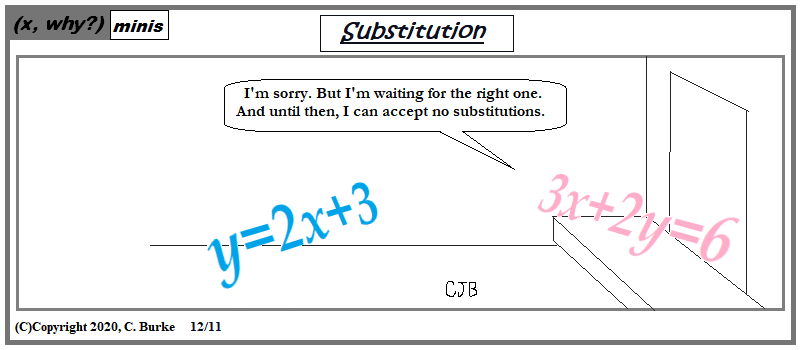(C)Copyright 2020, C. Burke. "AnthroNumerics" is a trademark of Christopher J. Burke and (x, why?).

Not sure if she's waiting for the x or the y to be one, but in the case, that x is a zero.

Not that one should ever be waiting on their x for anything.

Come back often for more funny math and geeky comics.## Thursday, December 10, 2020

### Circumference is 2 Pi r

(Click on the comic if you can't see the full image.)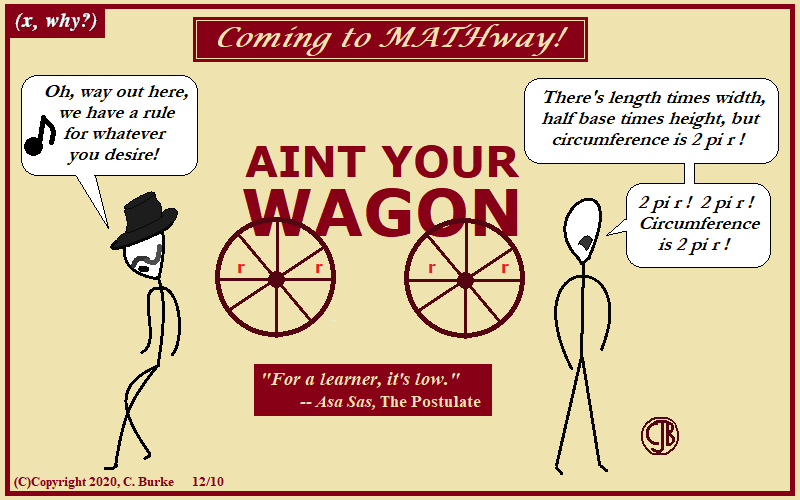(C)Copyright 2020, C. Burke. "AnthroNumerics" is a trademark of Christopher J. Burke and (x, why?).

It begs the question: whose wagon is it? (And what is all she wants for Christmas?)

The song came to me quite a while ago, along with the critic pun, which was originally supposed to be the after text.

I don't remember when "Aint" came to mind, but I knew I couldn't put that in the title. So I went with the formula. Oddly, there weren't too many that fit well into this song.

In any case, I'm sure a Western math musical would make many want to travel Eastward.

Update: I found my notes from a while ago. My original title was "Paint Your Numbers", but I'd forgotten that. Of course, that means I can use that for a different gag.

Come back often for more funny math and geeky comics.## Wednesday, December 09, 2020

### STAAR (State of Texas Assessments of Academic Readiness) Algebra I, May 2018, cont.

More STAAR problems.

Read each question carefully. For a multiple-choice question, determine the best answer to the question from the four answer choices provided. For a griddable question, determine the best answer to the question.

51. What is the slope of the line that passes through the points (5, −11) and (−9, 17)?

A -2
B -1/2
C 7
D 2

Change in y divided by change in x

(17 - -11) / (-9 - 5) = 28 / -14 = -2

52. What is the x-value of the solution to this system of equations?

x = 2y − 4
7x + 5y = −66

F -2
G -19/7
H -8
J -62/19

Substitute (2y - 4) for x in the second equation

7(2y - 4) + 5y = -66
14y - 28 + 5y = -66
19y = -38
y = -2

An odd coincidence that two answers in a row were the first choice, which was -2.

53. Which expression is equivalent to SQRT(96)?

A 24
B 8 SRT(6)
C 48
D 4 SQRT(6)

96 is not a perfect square, so it will not have an integer square root. Choices A and C are eliminated.
The factors of 96 are 2 x 2 x 2 x 2 x 2 x 3. (I got that by dividing 96 by 2 repeatedly until I only had 3 remaining.
Group the factors into pairs: SQRT( (2x2) x (2x2) x 2 x 3 )
That equals 2 x 2 x SQRT( 2 x 3 ), which is 4 SQRT(6).

54. Which line appears to have an x-intercept of -5 and a y-intercept of 3?

An x-intercept of -5 means the point (-5, 0) is on the line. A y-intercept of 3 means the point (0, 3) is on the line.

The graph that shows this is Choice G.

Choice J flips those numbers.

Choice F flips and negates those numbers.

Choice H negates those numbers.

END OF EXAM.

More to come. Comments and questions welcome.

More STAAR problems.

## Tuesday, December 08, 2020

### Perpendiculous

(Click on the comic if you can't see the full image.)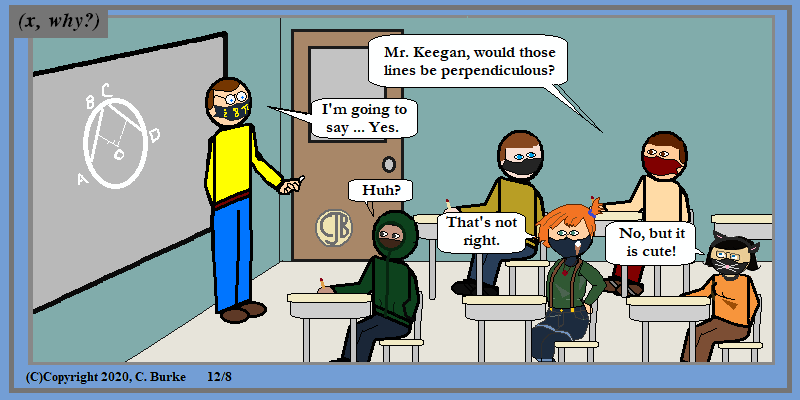(C)Copyright 2020, C. Burke. "AnthroNumerics" is a trademark of Christopher J. Burke and (x, why?).

If he walked around with his arms sticking straight out from his body then Aristophanes would be perpendiculous!

It's a silly thing students say. Or that teachers may say when they have too many things running through their heads at the same time.

But Missy is incorrect. Those angles are definitely right if they're "perpendiculous".

Looking at the students, I don't think I gave the one on the top left a name yet. The rest, going clockwise, are Shawn (misspelled Shaun sometimes), Isabel, Missy (aka Melissa), and Mark (with a hoodie covering his usual "42" shirt).

Note to admin: there is an Objective for the day displayed, but it's off the left side of the board. Yeah, that's the ticket.

I'm starting to get playful with the facemasks because they are otherwise boring and expressionless. Oddly, in real life, I prefer them plain or simple in pattern. I don't mind anyone celebrating their hobbies or fandoms, but I consider masks a necessary evil for the time being. I look forward to no longer needing them, and won't spend money on "stylized" ones. A year from now, I hope to wear last year's fandom T-shirt and ballcap without last year's facemask. End of rant.

Come back often for more funny math and geeky comics.### STAAR (State of Texas Assessments of Academic Readiness) Algebra I, May 2018, cont.

More STAAR problems.

Read each question carefully. For a multiple-choice question, determine the best answer to the question from the four answer choices provided. For a griddable question, determine the best answer to the question.

46. Scientists are studying a bacteria sample. The function f(x) = 245(1.12)x gives the number of bacteria in the sample at the end of x days.

Which statement is the best interpretation of one of the values in this function?

F The initial number of bacteria is 12.
G The initial number of bacteria decreases at a rate of 88% each day.
H The number of bacteria increases at a rate of 12% each day.
J The number of bacteria at the end of one day is 245.

Answer: H The number of bacteria increases at a rate of 12% each day.
Taking apart the function: 245 is the initial number, and 1.12 indicates a 12% increase.

The initial number is not 12. The bacteria are increasing, not decreasing. The starting value is 245, not the amount after 1 day.

47. The daily cost of hiring a plumber, y, to work x hours on a repair project can be modeled using a linear function. The plumber charges a fixed cost of \$80 plus an additional cost of \$45 per hour. The plumber works a maximum of 8 hours per day.

For one day of work, what is the range of the function for this situation?

A 0 ≤ x ≤ 8
B 80 ≤ y ≤ 440
C 0 ≤ x ≤ 10
D 45 ≤ y ≤ 685

Answer: B 80 ≤ y ≤ 440
The plumber charges \$80 before any hours of work have been done. This will be the minimum charge. Only one choice, B, has this as the minimum.

Choice A refers to the amount of hours he might work. Choice C doesn't seem to make any sense. Choice D has the equation backward with a fixed \$45 and \$80 per hour.

48. The graph of g(x) = x2 was transformed to create the graph of h(x) = -(x/4)2. Which of these describes the transformation from the graph of g to the graph of h?

F A reflection over the x-axis and a horizontal stretch
G A reflection over the y-axis and a horizontal stretch
H A reflection over the x-axis and a vertical stretch
J A reflection over the y-axis and a vertical stretch

Answer: F A reflection over the x-axis and a horizontal stretch
You can put both of these in your graphing calculator and just look at them.

The negative coefficient means that the parabola means that it will be flipped upside down, reflected across the x-axis. The 1/4 (which will become 1/16 when it's squared) will flatten out the curve, which is to say that is will be stretched out horizontally.

49. Which expression is equivalent to

(10q2w7) / (2w3) X (4(q6)2) / (w-5)

for all values of q and w where the expression is defined?

A (32q7) / w
B 20q17w9
C 32q7w9
D 20qw

In the first fraction, simplify 10/2 to get 5. Simplify w7 / w3 to get w4.

In the second fraction, (q6)2 becomes q12. Also, w-5 in the denominator becomes w5 in the numerator.

At this point, there is nothing left in the denominator.

In the numerator, there is (5)(4) = 20, w4 * w5 = w9, q5 * q12 = q17.

50. A graph of a quadratic function is shown on the grid.

Which coordinates best represent the vertex of the graph?

F (2.4, 0)
G (0, −1)
H (−0.4, 0)
J (1, −2)

The vertex is that minimum point at the bottom of the curve. The x-coordinate is 1, and the y-coordinate is -2. (1, -2).

Choices F and H appear to be the zeroes (roots) of the function. Choice G is the y-intercept, which is rarely important with quadratic functions (in my own opinion).

More to come. Comments and questions welcome.

More STAAR problems.

## Monday, December 07, 2020

### STAAR (State of Texas Assessments of Academic Readiness) Algebra I, May 2018, cont.

More STAAR problems.

Read each question carefully. For a multiple-choice question, determine the best answer to the question from the four answer choices provided. For a griddable question, determine the best answer to the question.

41. The area of a rectangular trampoline is 112 ft2. The length of the trampoline is 6 ft greater than the width of the trampoline. This situation can be represented by the equation w2 + 6w − 112 = 0.

What is the width of the trampoline in feet?

A 7 ft
B 16 ft
C 8 ft
D 14 ft

If the length X the width of a rectangle is 112 ft2, what are the possible lengths and widths? Hint: They have to have a difference of 6 because the length is 6 more than the width.

The factors of 112 are (1, 112), (2, 56), (4, 28), (7, 16), (8, 14). The last pair has a difference of 6. The width is 8 and the length is 14.

42. The table shows the linear relationship between the balance of a student’s savings account and the number of weeks he has been saving.

Based on the table, what was the rate of change of the balance of the student’s savings account in dollars and cents per week?

It's a linear relationship, so any two points can give you the rate of change. As always, if I have a chance to use 0 or 1, I'll take it. If I can use smaller numbers instead of bigger numbers, I'll do that, too.

Rate of change: (39 - 32) / (1 - 0) = 7 / 1 = 7
\$7.00 per week.

43. The graph of a linear function is shown on the grid.

Which equation is best represented by this graph?

A y + 7 = −3(x − 4)
B y + 1 = −3(x + 2)
C y − 4 = 3(x + 7)
D y − 2 = 3(x − 1)

Answer: A y + 7 = −3(x − 4)
The slope is negative, so choices C and D are eliminated.

According to the other choices, either (4, -7) or (-2, -1) should be a point on the graph. We can see that (4, -7) is on the graph, but (-2, -1) is not. In fact, the graph doesn't even go through any part of Quadrant III.

44. Which expression is a factor of 9r2 − 4r + 1?

F 3r − 1
G r − 1
H 9r − 1
J There are no real factors.

Answer: J There are no real factors.
What are two factors of (9)(1) that add up to -4? Answer: there are none.

If you graph this function, you will see that it doesn't cross the x-axis.

45. The graph of 2x − 5y = 10 is shown on the grid.

Which ordered pair is in the solution set of 2x − 5y ≥ 10?
A (0, 5)
B (5, 0)
C (−2, 5)
D (−5, 2)

Look at the point (0, 0). Is 2(0) - 5(0) ≥ 10? Is 0 - 0 ≥ 10? Obviously not. So (0, 0) is not is the solution set. Therefore, the solution is not above the line, but below the line. So which of these points is pn or below the line?

The other way to look at it is this: Plot the four points. Choice A is above the line. Choice B is on the line. Choice C is above the line. Choice D is above the line. Three of the choices are above the line, which means that they are ALL solutions or NONE of them are solutions. Since they can't all be, then none of them are. The answer is B.

More to come. Comments and questions welcome.

More STAAR problems.

## Friday, December 04, 2020

### STAAR (State of Texas Assessments of Academic Readiness) Algebra I, May 2018, cont.

More STAAR problems.

Read each question carefully. For a multiple-choice question, determine the best answer to the question from the four answer choices provided. For a griddable question, determine the best answer to the question.

36. Which expression is equivalent to (h2 + 9h − 1)(−4h + 3)?

F −4h3 − 33h2 + 31h − 3
G 4h3 + 39h2 − 23h − 3
H −4h3 − 39h2 + 23h + 3
J 4h3 + 33h2 − 31h + 3

Answer: F −4h3 − 33h2 + 31h − 3
It should be obvious that the leading term will be the product of h2 and -4h, which will be -4h3. That eliminates G and J.

Likewise, the final term is the product of (-1) and (3), which is -3, which eliminates H.

If you needed to work it out, do the following:

(h2 + 9h − 1)(−4h + 3)
-4h3 + 3h2 - 36h2 + 27h + 4h - 3
-4h3 - 33h2 + 31h - 3

37. There are 15 plates in a kitchen cabinet. The diameter of each plate is either 7 inches or 12 inches. The diameter of all 15 plates combined is 140 inches.

Which system of equations can be used to find the number of 7-inch plates, x, and the number of 12-inch plates, y, that are in the cabinet?

A x + y = 140 ; 12x + 7y = 15
B 7x + 12y = 140 ; 7x + 12y = 15
C x + y = 15 ; 7x + 12y = 140
D x + y = 15 ; 12x + 7y = 140

Answer: C x + y = 15 ; 7x + 12y = 140
The total number of plates is 15, so x + y = 15. Eliminate A and B.

The variable x is defined to be the 7-inch plates, so the total number of inches in diamter is 7x, not 12x. Likewise, the number of 12-inch plates is y, so we need 12y.

If you were solving this problem on your own, and the variables had not been pre-defined for you, then there would be no difference in using either C or D to solve the problem, as long as you knew which varaible stood for which object.

Choice A is backward with its 140 and 15 -- 140 dishes have a combined diameter of 15 inches? It must be a miniatures collection, or toys in a tiny doll house. Choice B has a pair of parallel lines, which has no solution.

38. The graph of quadratic function f is shown on the grid.

Which of these best represents the domain of f ?

F −3 ≤ x ≤ 2
G All real numbers
H y ≥ 5.5
J All real numbers less than −3 or greater than 2

Domain is the valid x values that can be input to the function. There are no numbers for which this quadratic is undefined. Just because only −3 ≤ x ≤ 2 is shown doesn't mean that that is the entirety of the graph. (Note the arrows at the ends of the line.)

Choice H would be correct for the Range. When you see something like this, always go back and reread the question. Make sure it said what you thought it said. (You'd be surprised at the number of times a person can misread something and instead read what they expected to see instead of what was there.)

39.What is the equation in slope-intercept form of the line that passes through the point (2, −2) and is perpendicular to the line represented by y = 2/5 x + 2?

A y = 5/2 x − 7
B y = 5/2 x + 7
C y = −5/2 x − 3
D y = −5/2 x + 3

Answer: D y = −5/2 x + 3
The slope of a line perpendicular to another line must be the inverse reciprocal of the slope of the original line. That is, the two slopes will have a product of -1.

The inverse reciprocal of 2/5 is -5/2. Eliminate A and B.

Substitue x = 2 into the other equations, and see which gives you -2.
y = -5/2 (2) - 3 = -5 - 3 = -8
y = -5/2 (2) + 3 = -5 + 3 = -2.

To work it out, if there weren't any choices, use slope-intercept form:

y - (-2) = -5/2 (x - 2)
y + 2 = -5/2 x + 5
y = -5/2 x + 3

40. Which graph best represents f(x) = 2(5)2?

When x = 0, 5x = 50 = 1, and 2(1) = 2.

When x = 1, 5x = 51 = 5, and 2(5) = 10.

The only graph with (0, 2) [approximately] and (1, 10) is graph F.

Choice J looks like f(x) = 5(2)x.

Choice H looks like f(x) = 10(2)x.

Choice G looks like f(x) = 5x.

More to come. Comments and questions welcome.

More STAAR problems.

### (x, why?) Mini: Pseudo-

(Click on the comic if you can't see the full image.)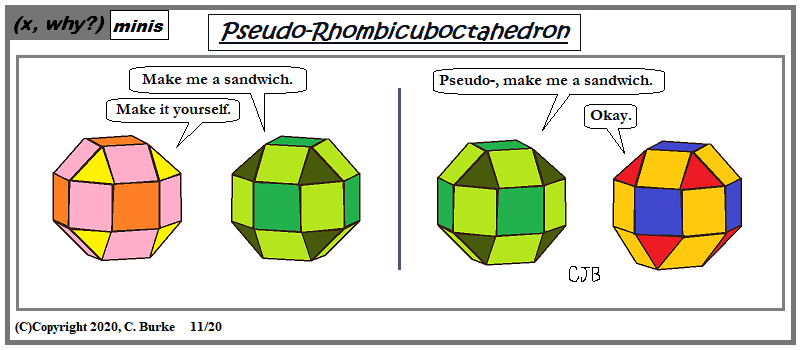(C)Copyright 2020, C. Burke. "AnthroNumerics" is a trademark of Christopher J. Burke and (x, why?).

One is derivative of another

In this case, this comic is derivative of two earlier ones, plus the more famous one from that other geeky, math/science/computer comic website. (You know which one.) I used a version of this when Leslie Nielsen passed away. And that time, I'd forgotten that I'd done an earlier version based on a Phil Collins song.

The "sudo" referenced here is the "sudo" computer command, which allows a user to run something with the privileges of another person (usu the "superuser"), even if they couldn't make the computer "do" it on their own.

As for the math, there is a tweet with a calendar problem for today from @GWOMaths about the Pseudo-Rhombicuboctahedron. If it was today's tweet, how did I know about it? Well, you might want to take a look at the answer tomorrow.

A Rhombicuboctahedron is an Archimedean solid with 26 faces: 18 squares and 8 equilateral triangles. A Pseudo-Rhombicuboctahedron is a similar object, but one section of it (top or bottom) is rotated by 45 degrees (1/8 of a full-rotations) or by some other odd multiple of 45. Being offset like this reduces some of the properties of the regular Rhombicuboctahedron.

The twisting is reminscent of the antiprism.

Come back often for more funny math and geeky comics.## Wednesday, December 02, 2020

### STAAR (State of Texas Assessments of Academic Readiness) Algebra I, May 2018, cont.

More STAAR problems.

Read each question carefully. For a multiple-choice question, determine the best answer to the question from the four answer choices provided. For a griddable question, determine the best answer to the question.

31.Which expression is a factor of 21x2 + 13x - 20?

A 3x − 4
B 7x − 5
C 7x + 4
D 3x + 5

What are the factors of (ac) that add up to b?

(21)(-20) = -420. What factors of -420 have a sum of +13.
If you start with, say, -10 and 42, which add up to 32, you can see that you need to get the numbers closer together. -14 and 30 is +16, which is closer. -15 and 28 gives you the result you're looking for.

Rewrite 21x2 + 13x - 20 as 21x2 - 15x + 28x - 20
Now separate them and factor: (21x2 - 15x) + (28x - 20)
3x(7x - 5) + 4(7x - 5)
= (3x + 4)(7x - 5)

32. What are the equation and slope of the line shown on the grid?

F y = 6; slope is -1/6.
G x = 6; slope is zero.
H y = 6; slope is 6.
J x = 6; slope is undefined.

Answer: J x = 6; slope is undefined.
The slope of a vertical line is undefined. It is not zero. A slope of zero would be a horizontal line.
Vertical lines have equations like x = a, where a is the coordinate of the x-axis. There is no y in the equation.

33. In the year 1900, the total number of metric tons of copper produced in the world was 495,000. Each year since 1900, the total number of metric tons of copper produced has increased on average by about 3.25% over the amount produced the previous year.

Which function models the total number of metric tons of copper produced in the year that is x years since 1900?

A c(x) = 495,000(1.0325)x
B c(x) = 495,000(0.9675)x
C c(x) = 495,000x1.0325
D c(x) = 495,000x0.9675

If the amount has increased, then the rate will be over 100%. An increase of 3.25% means that the rate is 103.25%, or 1.0325, which is Choice A.

Choice B represents a 3.25% reduction every year. Choices C and D are meaningless nonsense, created by mixing up the elements of the function.

34. What is the solution to 34x + 95 = 3(14x + 9)?

34x + 95 = 3(14x + 9)
34x + 95 = 42x + 27
95 = 8x + 27
68 = 8x
8.5 = x

35. The graphs of linear functions f and g are shown on the grid.
Which function is best represented by the graph of g?

A g(x) = f(x) - 4
B g(x) = 1/3 f(x)
C g(x) = f(x) - 2
D g(x) = 3f(x)

Answer: B g(x) = 1/3 f(x)
This is an interesting problem for me because it's not something that we encounter in New York in Algebra 1. In New York, when discussing transformations of functions, the focus is on translations, rigid motions, not dilations.

The first thing you notice about the graph is that the slope has changed, and the two lines intersect. This cannot happen in a translation, so Choices A and C are eliminated.

The new function has a smaller (less steep) slope than the original. Even without checking the points, it should be obvious that the slope must be 1/3 and not 3. If you check the graph, you can see that f(3) = 3 and g(3) = 1, f(6) = 6 and g(6) = 2. g(x) is 1/3 of f(x).

More to come. Comments and questions welcome.

More STAAR problems.

## Tuesday, December 01, 2020

(Click on the comic if you can't see the full image.)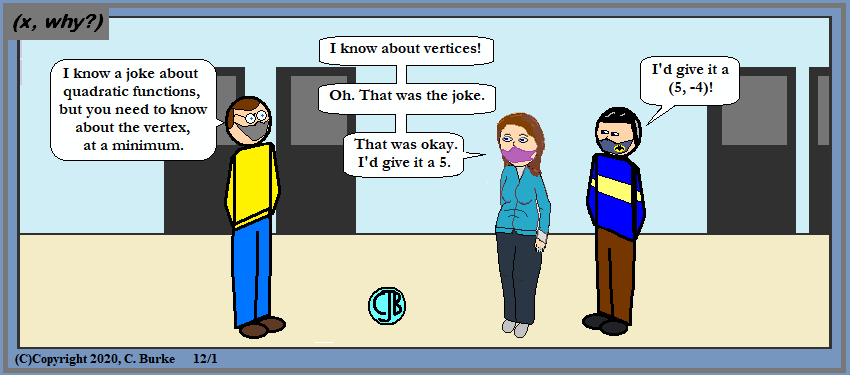(C)Copyright 2020, C. Burke. "AnthroNumerics" is a trademark of Christopher J. Burke and (x, why?).

A five is nothing. Watch out for the zeroes!

If Ken is to be believed (never a sure bet), then the function would be in the form of y = a(x - 5)2 - 4. Since we don't know the zeroes, we don't know what a is. Conversely, since we don't know a, we don't know the zeroes. However, if a = 1, then the zeroes are x = 3 and x = 7.

Come back often for more funny math and geeky comics.### STAAR (State of Texas Assessments of Academic Readiness) Algebra I, May 2018, cont.

More STAAR problems.

Read each question carefully. For a multiple-choice question, determine the best answer to the question from the four answer choices provided. For a griddable question, determine the best answer to the question.

26.Which function is equivalent to f(x) = -4(x + 7)2 - 6?

F f(x) = −4x2 − 56x − 202
G f(x) = −4x2 + 14x + 43
H f(x) = −4x2 − 56x − 172
J f(x) = −4x2 + 190

Answer: F f(x) = −4x2 − 56x − 202
Look at the constant terms in the four choices. They are all different. Two of them make no sense at all.

The final term has to be (7)2 X (-4) - 6. That cannot be a positive number, so G and J are eliminated.
(7)2 X (-4) - 6 = -202. So it is Choice F.

Working it out would give you:

f(x) = -4(x + 7)2 - 6
= -4(x2 + 14x + 49) - 6
= -4x2 - 56x - 196 - 6
= -4x2 - 56x - 202

22. A paper airplane was thrown from the top of a tall building. The height of the paper airplane above the ground can be found using the function y = −1.5x + 60, where x is the time in seconds the airplane has been in the air.
How many seconds did it take the paper airplane to reach the ground?

The graph isn't necessary. In fact, graphing a slope of -1.5 when the scale is 5 might even be confusing. (It's actually the same as if the scale was 1, as far as counting boxes goes.)

0 = -1.5x + 60
-60 = -1.5x
40 = x

After 40 seconds the airplane will reach the ground.

28. Which expression is equivalent to (q4)-3/q-15

F q27
G 1/q27
H q3
J 1/q3

(q4)-3/q-15 = q(4)(-3) - (-15) = q3

29. Which graph best represents a quadratic function that has only one zero?

A quadratic function with only one zero only touches the x-axis once. That means that the vertex must be on the x-axis itself as either a minimum or a maximum. That is choice B.

Choices A and C have two zeroes. Choice D has no zeroes. (Zero zeroes if you will.)

30. What is the solution set for −4x + 10 ≥ 5x + 55?

F x ≥ 5
G x ≥ 45
H x ≤ −5
J x ≤ −45

−4x + 10 ≥ 5x + 55
−9x + 10 ≥ + 55
−9x ≥ 45
x ≤ −5

Don't forget to flip the inequality symbol around.

More to come. Comments and questions welcome.

More STAAR problems.

## Monday, November 30, 2020

### STAAR (State of Texas Assessments of Academic Readiness) Algebra I, May 2018, cont.

More STAAR problems.

Read each question carefully. For a multiple-choice question, determine the best answer to the question from the four answer choices provided. For a griddable question, determine the best answer to the question.

21. The graph shows the linear relationship between the maximum area in square feet that can be painted and the number of gallons of paint used.

Which of these best represents the rate of change of the maximum area painted with respect to the number of gallons of paint used?

A 200 ft2/gal
B 1/200 ft2/gal
C 400 ft2/gal
D 1/400 ft2/gal

If you go to 1 gallon on the x-axis, that lines up with 400 ft2 on the y-axis. Change in y / Change in x, it is 400 ft2 / gal.

22. What are the solutions to (x + 7)2 = 81?

F -74 and 88
G -2 and 16
H -88 and 74
J -16 and 2

Take the square root of both sides: x + 7 = + 9
Split and solve: x + 7 = 9 or x + 7 = -9
x = 2 or x = -16

You could also have plugged in 2 for x and saw that it was a correct solution, while all of incorrect choices would be way off.

23. What appears to be the domain of the part of the exponential function graphed on the grid?

A -1 < x < 3
B -1 < y < 3
C 5.3 < x < 27
D 5.3 < y < 27

Answer: A -1 < x < 3
This gets repeated many times, but the domain is the x values. Eliminate Choice B and D.

The leftmost point has an x coordinate of -1, so the correct choice is A.

Choices B and C are just nonsense.

24. The tables of ordered pairs represent some points on the graph of lines q and v.

Which system of equations is represented by lines q and v?

F 21x − y = 9 ; 5x + 6y = 40
G 3x − y − = −27 ; x + 2y = 16
H 21x −y = 9 ; 5x + 6y = 20
J 9x − y = −27 ; x + 2y = 8

Answer: G 3x − y = −27 ; x + 2y = 16
Working backward from the answers is the quickest route. You could create the linear equations for each table, but then you would have to rewrite them in Standard form to match the choices.

My preference is to work with 0 when it is available. Looking at the table for line q, we see the point (-9, 0). Therefore x = -9 and y = 0 is a solution to one of those equations. (Note that it doesn't have to be the first of the pair. Nothing says that it is.)

21(9) - 0 =/= 9. 5(-9) + 0 =/= 40.
3(-9) - 0 = -27. (Choice G looks good so far).
21(9) - 0 =/= 0. 5(-9) + 0 =/= 20.
9(-9) - 0 =/= -27. -9 + 0 =/= 8.

Only Choice G is possible. Check the second equation for (0, 8). 0 + 2(8) = 16. Check!

25. Which situation does NOT show causation?

A When the student population at a school increases, the number of teachers at the school increases.
B When the amount of sugar in a quart of apple juice is reduced, there are fewer calories in each serving.
C When there are more workers on a project, the project is completed in less time.
D When there is more protein in an athlete’s diet, the athlete scores more points in a game.

Answer: D When there is more protein in an athlete’s diet, the athlete scores more points in a game.

If you get more students, then you need more teachers. One causes the other.

If you reduce the sugar, you reduce the number of calories.

If you put more workers on a project, it gets completed in less time. (There's a point of diminishing returns, but that's a little beyond the level of this question. Also, there's a joke about the project being a symphony -- a bigger orchestra won't complete it sooner. But this is more about "man-hours" to finish.)

There are many factors in athletic performance. Is increasing protein one of them? It doesn't show causation. Maybe if said what the protein did, or how that affected performance, you might show causation. But this itself does not.

More to come. Comments and questions welcome.

More STAAR problems.

## Sunday, November 29, 2020

### STAAR (State of Texas Assessments of Academic Readiness) Algebra I, May 2018, cont.

More STAAR problems.

Read each question carefully. For a multiple-choice question, determine the best answer to the question from the four answer choices provided. For a griddable question, determine the best answer to the question.

16. The water level of a river was measured each day during a two-week period. The graph models the linear relationship between the water level of the river in feet and the number of days the water level was measured.

Which statement best describe the y-intercept of the graph?

F The water level increased by 0.25 ft per day.
G The maximum water level was 19.5 ft.
H The initial water level was 16 ft.
J The water level was measured for 14 days.

Answer: H The initial water level was 16 ft.
The y-intercept represents the initial amount in a graph or fuction.

The other statements are all true, but have nothing to do with the y-intercept. In F, the incease in water level per day is the slope. In G, the maximum water level is a part on the graph, but not the initial point. In J, we've established the domain, 0 - 14, which concerns the x values, not the y values.

17. There are 1,024 players in a tennis tournament. In each round, half the players are eliminated. Which function can be used to find the number of players remaining in the tournament at the end of x rounds?

A f(x) = 1,024(1.50)x
B f(x) = 1,024(0.50)x
C f(x) = 1,024(1.05)x
D f(x) = 1,024(0.05)x

If half the teams are eliminated, then the rate of decay is .50, or 50%.

Choice A shows a 50% increase, meaning more players are joining the tournament. Choice C shows a 5% increase. Choice D shows a 95% decrease, with only 5% advancing to the next round.

18. Which statement about g(x) = x2 − 576 is true?

F The zeros, −288 and 288, can be found when 0 = (x + 288)(x − 288).
G The only zero, 288, can be found when 0 = (x − 288)2.
H The zeros, −24 and 24, can be found when 0 = (x + 24)(x − 24).
J The only zero, 24, can be found when 0 = (x − 24)2.

Answer: H The zeros, −24 and 24, can be found when 0 = (x + 24)(x − 24).
The Difference of Squares rule states that x2 - n2 can be factored into (x + n)(x - n). The "inner" and "outer" terms when multiplied form a zero pair and cancel out.

In this problem, 576 represents n2, so n is SQRT(576), which is 24, not 288.

19. Which graph best represents the solution set -4x < 6y - 54?

Two questions to ask yourself: Is it a solid or a broken line? Is (0, 0) part of the solution?

Since we want "less than or equal to" (<) and not just "less than" (<), the graph needs to have a solid line, so Choices B and C are eliminated.
Substitute (0, 0) and we get 0 < 0 - 54, which is not a true statement. Therefore, (0, 0) is not a part of the solution, and that side of the line cannot be shaded. Choice D is eliminated.

The longer method involves rewriting the problem into slope-intercept form.

-4x < 6y - 54
6y - 54 > -4x
6y > -4x + 54
y > -4/6 x + 9

At this point, you can see that the line needs to be shaded above, not before. Also, you can see that choice A have a slope of -4/6 (or -2/3), while D has a slope of -6/4 (or -3/2).

20.Given f(x) = 1/3 (4 - x)2, what is the value of f(16)?

f(16) = 1/3 (4 - 16)2
= 1/3 (-12) 2
= 1/3 (144)
= 48

More to come. Comments and questions welcome.

More STAAR problems.

## Friday, November 27, 2020

### STAAR (State of Texas Assessments of Academic Readiness) Algebra I, May 2018, cont.

More STAAR problems.

Read each question carefully. For a multiple-choice question, determine the best answer to the question from the four answer choices provided. For a griddable question, determine the best answer to the question.

11. Which expression is equivalent to x2 − 17x − 60?

A (x - 20)(x + 3)
B (x - 5)(x - 12)
C (x - 20)(x - 3)
D (x + 5)(x - 12)

Answer: A (x - 20)(x + 3)
The minus sign in front of the 60 tells you that one factor is positive and one factor is negative. That eliminates Choices B and C.

What are two factors of -60 that add up to -17? -20 + 3 = -17, Choice A is the answer.

12. A zookeeper recorded the feeding schedule for a baby rhinoceros for 20 weeks. The table and scatterplot show the percentage of the baby rhinoceros’s body mass that was used to determine the amount of food given at each feeding as a linear function of its age in weeks.

What is the best prediction of the percentage of the baby rhinoceros’s body mass that should be used to determine the amount of food given at each feeding when it is 25 weeks old?

F 8.5%
G 6%
H 2.5%
J 10%

Put the data into your graphing calculator and do a linear regression. You will get a = -.5448... and b = 19.80...
That makes your line of best fit, rounded to the nearest hundredth, y = -.54x + 19.80.
Substitute 25 for x and you get y = -.54(25) + 19.80 = 6.3, which is approximately 6%, which is Choice G.

13. What are the domain and range of f(x) = −37?

A Domain: All real numbers greater than or equal to −37; Range: All real numbers
B Domain: {−37} ; Range: All real numbers
C Domain: All real numbers ; Range: All real numbers greater than or equal to −37
D Domain: All real numbers ; Range: {−37}

Answer: D Domain: All real numbers ; Range: {−37}
The range is {-37}. No matter what the input is, the output is -37. That is the entirety of the range. That gives you exactly one option, Choice D.

The input is All real numbers. It doesn't matter what x is, and there are no restrictions on it. There are no values that are undefined.

14. The graph of quadratic function p is shown on the grid.

If k(x) = x2 and p(x) = k(x) + n, what is the value of n? Record your answer and fill in the bubbles on your answer document.

The graph of the parent function k(x) = x2 goes through the origin, (0, 0). The illustrated graph of p(x) has its vertex at (0, -6). Therefore, p(x) = x2 - 6, which means that n = -6.

You can check this by substituting x = -3 or x = 3. Both numbers squared give 9 which after subtracting 6 becomes 3.

15. Two customers went to a post office to buy postcards and large envelopes. Each postcard costs the same amount, and each large envelope costs the same amount.

• • The first customer paid \$12 for 14 postcards and 5 large envelopes.
• • The second customer paid \$24.80 for 10 postcards and 15 large envelopes.

What was the cost in dollars of each large envelope?

A \$1.42
B \$0.35
C \$1.15
D \$0.63

The system of equations is as follows:

14x + 5y = 12
10x + 15y = 24.80
multiply the first equation by 3, and then subtract
42x + 15y = 36.00
10x + 15y = 24.80
32x = 11.20
x = 0.35, which is the cost of a postcard
14(.35) + 5y = 12
4.90 + 5y = 12
5y = 7.10
y = 1.42, which is the cost of a large envelope

More to come. Comments and questions welcome.

More STAAR problems.

## Thursday, November 26, 2020

### Happy Thanksgiving 2020!

(Click on the comic if you can't see the full image.)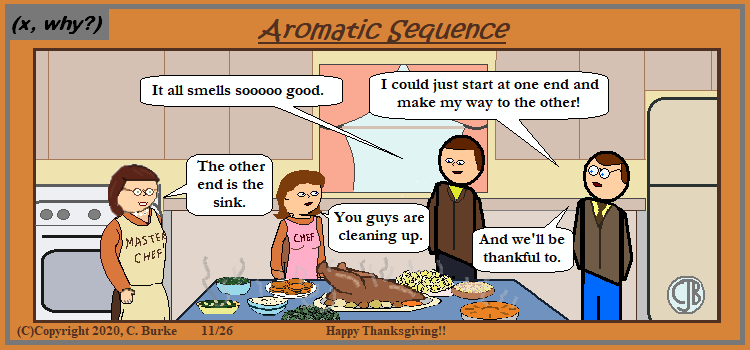(C)Copyright 2020, C. Burke. "AnthroNumerics" is a trademark of Christopher J. Burke and (x, why?).

From start to finish, there's a strong aromatic sequence from one course to the next.

A lot of food for one small family of four, but how many people can cook smaller Thanksgiving dinners and still call it a Thanksgiving dinner? I mean, what are you going to leave out?

And you know that there will be "food runs" to the relatives who couldn't be there.

Happy Thanksgiving from (x, why?)!

Come back often for more funny math and geeky comics.## Wednesday, November 25, 2020

### Call Me Mike

(Click on the comic if you can't see the full image.)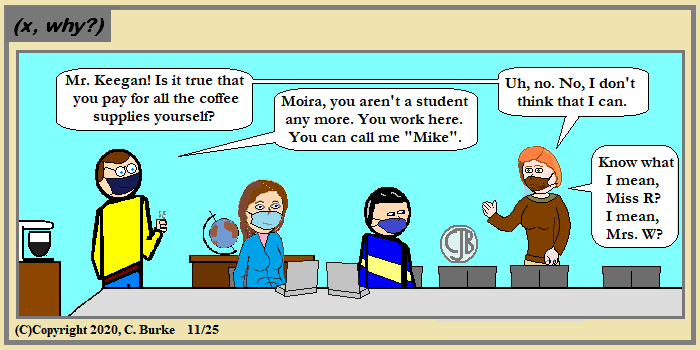(C)Copyright 2020, C. Burke. "AnthroNumerics" is a trademark of Christopher J. Burke and (x, why?).

It just sounds wrong, right?

Students who keep in touch on social media still call me Mr. B, which is fine. But I've seen a couple socially, and they've tried not calling me that, and it doesn't work for them. Even though people younger than them have no problem.

This, of course, carries over to all of my parents' friends. Keep in mind, I'm older than Mike.

On a comic note: Moira (first time mentioning a name) was a student here when she was younger. She became a teaching student of Michele's shortly after the wedding.

Come back often for more funny math and geeky comics.